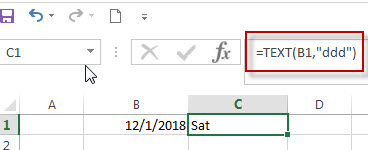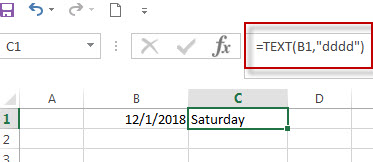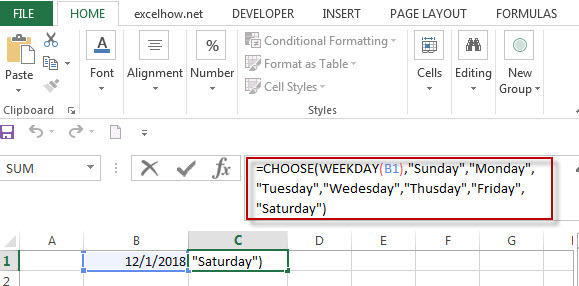# Convert Date to Day of Week in Excel (get day name from date )

In excel, how to get the day of week from an excel date value. How to return a day name of the week from a date that is contained in a cell in Microsoft Excel. This post will guide you how to convert date to day of week only using Text Function in Microsoft Excel.

## Convert date to day of week with Text Function (get day of week from date)

If you want to get the day name from a date in excel, you can use the TEXT function with a specified format code like “ddd” or “dddd”. We can try to use the below TEXT formula in excel:

```=TEXT(date,"ddd")
=TEXT(date, "dddd")```

If you want to get the day name of the week as an abbreviation from a date, such as: Mon, Tue, etc. Enter the following TEXT formula in the Cell C1 that you want to get the day name of the week, and then press ENTER:

`=TEXT(B1,"ddd")`If you want to get the full day name of the week, such as: Monday, Tuesday, etc. Enter the following TEXT formula in the Cell C1 that you want to get the day name of the week, then press ENTER:

`=TEXT(B1,"dddd")`## Get a Day Name of the Week Using WEEKDAY and CHOOSE function

The WEEKDAY function will get a day number of the week form a date.

We can use the returned value of WEEKDAY function as the first argument within the CHOOSE function. The CHOOSE function will use the returned weekday number as the first argument to return the nth day name from a list of day name.

So we can use the following CHOOSE function in combination with WEEKDAY function to get the full day name of the week from a date.

`=CHOOSE(WEEKDAY(B1),"Sunday","Monday","Tuesday","Wedesday","Thusday","Friday","Saturday")`For more format codes in excel formatting, you can refer to the below table:

 Format Code Description Examples 0 only display digits in its place #.00 – Forces the function to display two decimal places =Text(34.234,”\$##.00″) result: \$34.23 # Display the placeholder =Text(4.527,”#.##) result: 4.53 . the position of Decimal Point =Text(342.2,”0.00″) result: 342.20 d Day of the month or day of week d- one or two digit number (1-31) dd- two digit number (01-31) ddd-abbreviated day of week (Mon to Sun) dddd-full name of day of week(Monday to Sunnday) =Text(TODAY(),”DDDD”) result: Monday m The Month of the Year m- one or two digit number mm-two digit number mmm-abbreviated month(Jan to Dec) mmmm-full name of month(January to December)) =Text(TODAY(),”MM/DD/YY”) result:11/06/17 y year yy-two digit representation of year(e.g.01,17) yyyy-four digit representation of year(e.g. 2001,2017) =Text(TODAY(),”MM/DD/YY”) result:11/06/17

### Related Formulas

• Excel Convert numbers to Text
The Text function will accept a numeric value as the first argument, then based on the format code in the second argument to convert the number to text. You can convert all the standard number formats such as: dates, times, currency to Text string in excel.…
• Convert date to month name with Text Function
If you want to convert the date to a month name, you can use the TEXT function with a specified format code like “mmm”in excel.  You can try to run the following TEXT formula:=TEXT(B1,”mmm”)
• Convert Date to text with Text Function in Excel
you can use TEXT function in excel to convert dates to text in a specific format code. For example, The TEXT function can use the following patterns, like as: “mm/dd/yyyy”, “yyyy/mm/dd”, etc.
• Convert date to month and year only in excel
If you want to convert the date to month and year only, you can use “yyyymm” format code within the TEXT function in excel, so you can write down the below TEXT formula:=TEXT(date,”yyyymm”)
• Convert date to month and day only in excel
If you want to convert the date (mm/dd/yyyy) to month and day only, you can use “mm dd” format code within the TEXT function in excel, so you can write down the below TEXT formula: =TEXT(date,”mm dd”)

### Related Functions

• Excel Text function
The Excel TEXT function converts a numeric value into text string with a specified format. The TEXT function is a build-in function in Microsoft Excel and it is categorized as a Text Function. The syntax of the TEXT function is as below: = TEXT (value, Format code)…
• Choose function in excel
The Excel CHOOSE function returns a value from a list of values. The CHOOSE function is a build-in function in Microsoft Excel and it is categorized as a Lookup and Reference Function.The syntax of the CHOOSE function is as below:=CHOOSE (index_num, value1,[value2],…)…
• Excel WEEKDAY function
The Excel WEEKDAY function returns a integer value representing the day fo the week for a given Excel date and the value is range from 1 to 7.The syntax of the WEEKDAY function is as below:=WEEKDAY (serial_number,[return_type])…